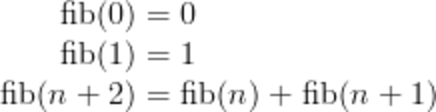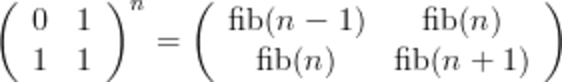# Re: Factor

Factor: the language, the theory, and the practice.

## Fibonacci Wars

Tuesday, May 17, 2011

Inspired by a recent article about the “worst algorithm in the world” for calculating Fibonacci numbers using Python, I wanted to show a few implementations in Factor.

### Recursive

Most people should be familiar with the simple definition for calculating the Fibonacci numbers:Some recursive implementations of this algorithm suffer from very slow performance due to the nature of hugely recursive functions, making them unsuitable for larger Fibonacci numbers. Thankfully, it is easy to implement caching using the memoize vocabulary:

``````MEMO: slow-fib ( m -- n )
dup 0 >= [ throw ] unless
dup 2 >= [
[ 2 - slow-fib ] [ 1 - slow-fib ] bi +
] when ;
``````

### Iterative

A better way would be to implement an iterative solution, that simply keeps the current and previous Fibonacci number on the stack, adding them the specified number of times:

``````: okay-fib ( m -- n )
dup 0 >= [ throw ] unless
[ 0 1 ] dip [ [ + ] [ drop ] 2bi ] times drop ;
``````

### Magical

A much better way (introduced by the original article) suggests taking advantage of a matrix expression for the Fibonacci numbers:Using this, we can implement a faster version in Factor (using locals to make it a bit more readable):

``````:: fast-fib ( m -- n )
m 0 >= [ m throw ] unless
m 2 >base [ CHAR: 1 = ] { } map-as :> bits
1 :> a! 0 :> b! 1 :> c!
bits [
[
a c + b *
b sq c sq +
] [
a sq b sq +
a c + b *
] if b! a! a b + c!
] each b ;
``````

### Performance

Some simple performance numbers show how much faster the “magical” version is, particularly for calculating large Fibonacci numbers (such as the the one-millionth number in the sequence).

10,000 100,000 1,000,000
`slow-fib` 0.0097 -- --
`okay-fib` 0.0053 0.2560 25.9608
`fast-fib` 0.0001 0.0076 0.4851

It’s worth pointing out that Factor is about as fast as Python 2.6 for the “magical” calculation. In Python 2.7 and later, certain improvements were made to their large number support, resulting in Python being about 3x faster.

However, if you want real performance, you can use C and the GNU Multiple Precision Arithmetic Library to implement the iterative algorithm (about 3 times faster than Factor), the magical algorithm (about 28 times faster than Factor), or use the builtin mpz_fib_ui function (about 44 times faster than Factor).

The code for this (as well as several version in C) are on my GitHub.